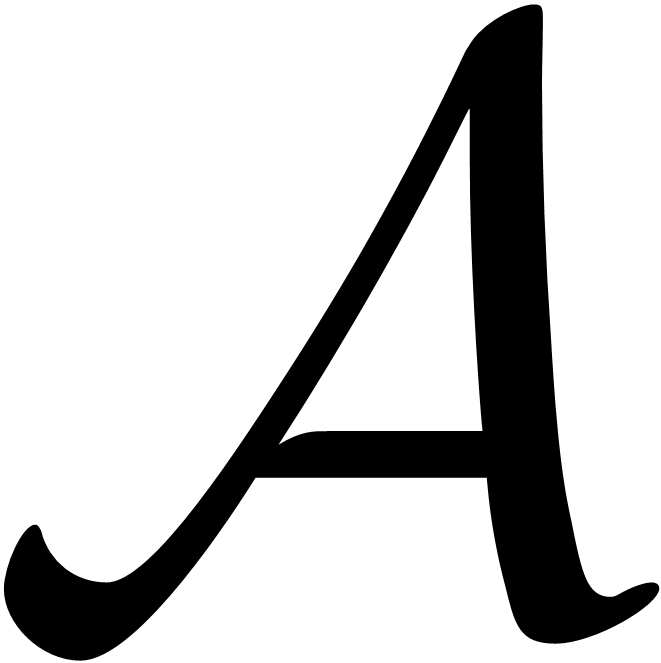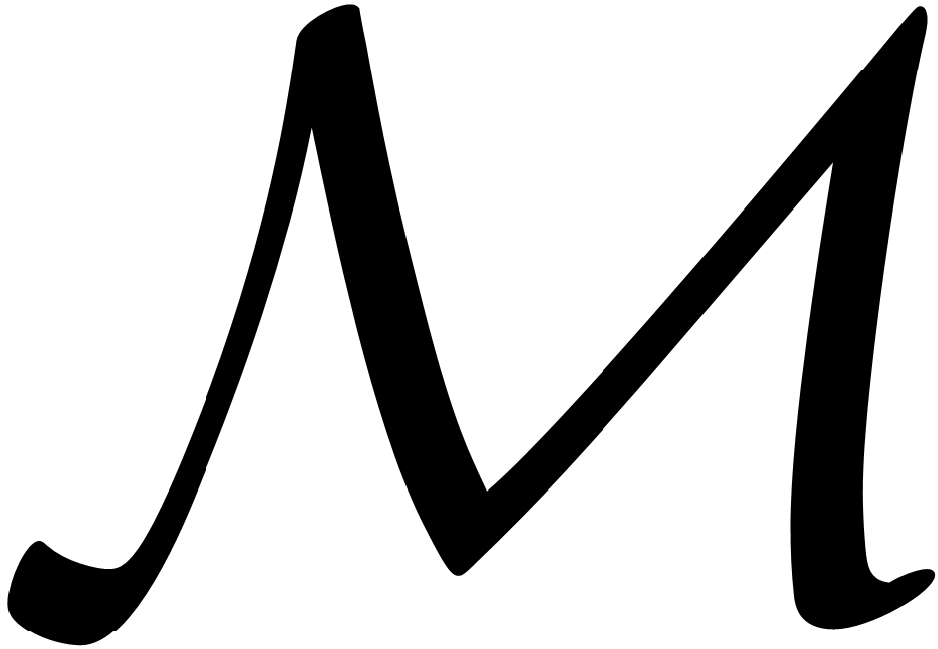Change code
ConfirmArchive
Special Issues
Characterizations of Jordan *-derivations on Banach *-algebras
Pure and Applied Mathematics Journal
Volume 9, Issue 5, October 2020, Pages: 96-100
Received: Aug. 11, 2020; Accepted: Sep. 18, 2020; Published: Oct. 28, 2020
Authors
Guangyu An, Department of Mathematics, Shaanxi University of Science and Technology, Xi’an, China
Ying Yao, Department of Mathematics, Shaanxi University of Science and Technology, Xi’an, China
Article ToolsAbstractPDF (206KB)
Abstract
Suppose thatis a real or complex unital Banach *-algebra,is a unital Banach-bimodule, and G ∈is a left separating point of. In this paper, we investigate whether the additive mapping δ:satisfies the condition A,B, AB = G ⇒ Aδ(B)+δ(A)B*= δ(G) characterize Jordan *-derivations. Initially, we prove that ifis a real unital C*-algebra and G = I is the unit element in, then δ (non-necessarily continuous) is a Jordan *-derivation. In addition, we prove that ifis a real unital C*-algebra and δ is continuous, then δ is a Jordan *-derivation. Finally, we show that ifis a complex factor von Neumann algebra and δ is linear, then δ (non-necessarily continuous) is equal to zero.
Keywords
Jordan *-derivation, Left Separating Point, C*-algebra, Factor
Guangyu An, Ying Yao, Characterizations of Jordan *-derivations on Banach *-algebras, Pure and Applied Mathematics Journal. Vol. 9, No. 5, 2020, pp. 96-100. doi: 10.11648/j.pamj.20200905.13
References

M. Breˇ sar and J. Vukman. On some additive mappings in rings with involution, Aequ. Math., 3 (1989), 178-185.

M. Breˇ sar and B. Zalar. On the structure of Jordan ∗-derivations, Colloq. Math., 63 (1992), 163-171.

Q. Chen, C. Li, X. Fang. Jordan (α,β)-derivations on CSL subalgebras of von Neumann algebras, Acta. Math. Sin., 60 (2017), 537-546.

S. Goldstein and A. Paszkiewicz, Linear combinations of projections in von Neumann algebras, Proc. Amer. Math. Soc., 116 (1992), 175-183.

J. He, J. Li, D. Zhao, Derivations, Local and 2-local derivations on some algebras of operators on Hilbert Csup>*-modules, Mediterr. J. Math., 14, 230 (2017).

S. Kurepa. Quadratic and sesquilinear functionals, Glasnik Mat. Fiz.-Astronom, 20 (1965), 79-92. 106 Guangyu An and Ying Yao: Characterizations of Jordan ∗-derivations on Banach ∗-algebras

L. Liu. 2-Local Lie derivations of nest subalgebras of factors, Linear and Multilinear algebra, 67 (2019), 448-455.

D. Liu, J. Zhang. Local Lie derivations on certain operator algebras, Ann. Funct. Anal., 8 (2017), 270-280.

X. Qi and X. Zhang. Characterizations of Jordan ∗- derivations by local action on rings with involution, Journal of Hyperstructures, 6 (2017), 120-127.

P. ˇSemrl. On Jordan ∗-derivations and an application,Colloq. Math., 59 (1990), 241-251.

P.ˇSemrl. Jordan ∗-derivations of standard operator algebras, Proc. Amer. Math. Soc., 120 (1994), 515-518.

P.ˇSemrl. On quadratic functionals, Bull. Austral. Math. Soc., 37 (1988), 27-28.

P. ˇSemrl. Quadratic functionals and Jordan ∗-derivations, Studia Math., 97 (1991), 157-165.

P. Vrbová, Quadratic functionals and bilinear forms, Casopis Pest. Mat., 98 (1973), 159-161.

J. Vukman, Some functional equations in Banach algebras and an application, Proc. Amer. Math. Soc., 100 (1987), 133-136.
PUBLICATION SERVICES